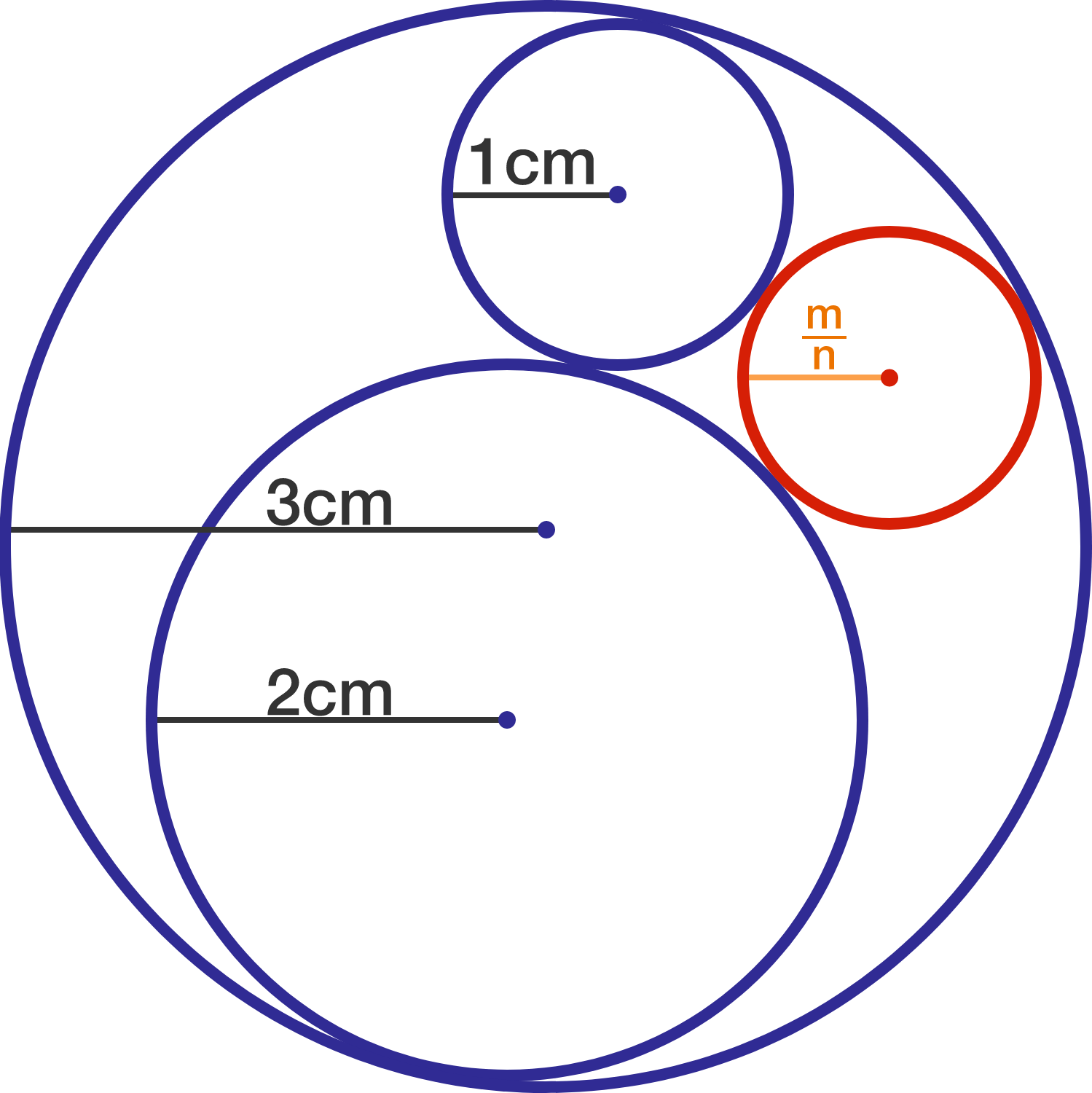# 4 Circles in Love

Geometry Level 5The diagram above shows 4 mutually tangent circles.

Their respective radii (in centimeters) in descending order of magnitude are 3, 2, 1, and $\frac{m}{n}$, where $m$ and $n$ are coprime positive integers. What is $m+n?$

×